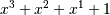# numpy.polyder¶

numpy.polyder(p, m=1)[source]

Return the derivative of the specified order of a polynomial.

Parameters: p : poly1d or sequence Polynomial to differentiate. A sequence is interpreted as polynomial coefficients, see poly1d. m : int, optional Order of differentiation (default: 1) der : poly1d A new polynomial representing the derivative.

polyint
Anti-derivative of a polynomial.
poly1d
Class for one-dimensional polynomials.

Examples

The derivative of the polynomialis:

```>>> p = np.poly1d([1,1,1,1])
>>> p2 = np.polyder(p)
>>> p2
poly1d([3, 2, 1])
```

which evaluates to:

```>>> p2(2.)
17.0
```

We can verify this, approximating the derivative with (f(x + h) - f(x))/h:

```>>> (p(2. + 0.001) - p(2.)) / 0.001
17.007000999997857
```

The fourth-order derivative of a 3rd-order polynomial is zero:

```>>> np.polyder(p, 2)
poly1d([6, 2])
>>> np.polyder(p, 3)
poly1d()
>>> np.polyder(p, 4)
poly1d([ 0.])
```

numpy.polyfit

numpy.polyint